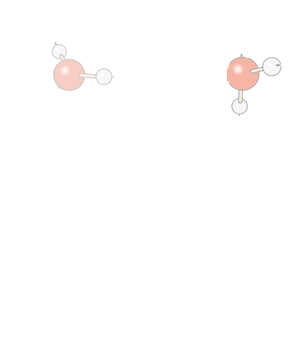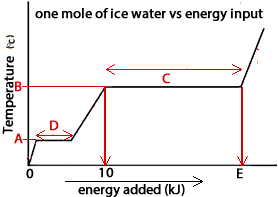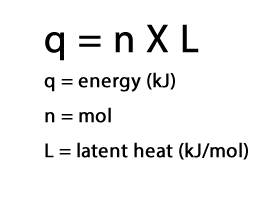Latent heat LATENT HEAT When an amount of substance changes state it absorbs or releases a certain amount of energy known as the latent heat. This amount of energy is fixed for a given quantity of substance . For example the latent heat of fusion of water, that is when ice melts to form liquid water, is about 334 joules per gram (J/g) or 6.0 kJmol-1. While the ice is absorbing energy and melting the temperature remains constant at 0oC. This is clearly shown with a phase-change diagram. How can that be? Energy is absorbed and goes into breaking strong hydrogen bonds between water molecules as opposed to increasing the average kinetic energy of the water molecules thus raising the temperature. Let's look at a few dot points. •Over the period of time that the phase change is taking place and latent heat is being absorbed, the temperature of a substance will not change. •The units for latent heat are kilojoules per mole (kJmol-1) and given the symbol "L". Depending on the phase change different terms apply. For example: •Latent heat of fusion- is used when the phase change is from solid to liquid and represents the energy absorbed to melt one mol of the substance.The temperature at this point is known as the melting temperature. •Latent heat of vaporisation- is used when the phase change is from liquid to gas and represents the energy absorbed to boil one mol of the substance. The temperature at this point is known as the boiling temperature •Latent heat of condensation - is used when the phase change from gas to liquid takes place and represents the energy releasd when one mol of the substance condenses. It happens to be exactly that of the latent heat of vaporisation but is given out rather than absorbed. Lets take one mol, or 18g, of solid water (ice) at 0oC. Supplying this amount of water with 6.0 kJ of energy, which happens to be the latent heat of fusion of water, disrupts all the hydrogen bonds that hold the water molecules in a tight crystal lattice. Now supplying a further 41 kJmol-1  when the temperature reaches 100 oC, which hapens to be the latent heat of vaporisation of water, completely breaks the hydrogen bonds between water molecules and causes the formation of gaseous water (steam).Consider the graph on the right of the temperature of one mol of solid water (ice) as it has energy supplied to it. 1) What is the value of A? 2) What is the value of B? 3) What is the value of E? 4) What section along the graph are hydrogen bonds between water molecules completely breaking? 5) What section along the graph are hydrogen bonds between water molecules being disrupted but still active? SolutionCalculations involving latent heat The heat energy required for a state change is given by the formula as shown on the right. Now let's see hwo to use this formula in calculations. ​ 1. Calculate the amount of heat energy, in kJ, required to evaporate 36.0g of water at 100oC given the latent heat of vaporisation of water is 41.0 kJmol-1. Step 1 Find the mol of water. => 36.0 / 18.0 = 2.00 mol Step 2 find the energy => q = n X L => energy = 2.00 X 41.0 kJ = 82.0 kJ